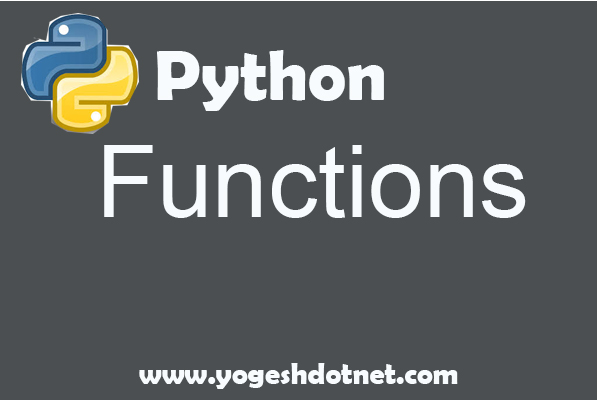functions in python - Tutorial

For Consultation : +91 9887575540

Stay Connected :functions in python

• Function definition
There are some simple rules to define a function in Python. They are as follows:
Use the def keyword followed by function name with parentheses ()
Any argument to the function must be placed within these parentheses ()
The code block must start with a colon :
The code within the function must be indented

def function_name(arguments):

return value

Function with variable length argument

There might be a scenario where you need to pass more arguments than specified during
the function definition. In this case, variable length arguments can be passed:

Pass by reference versus pass by value

Pass by reference is the term used in some programming languages, where values to the
argument of the function are passed by reference, that is, the address of the variable is
passed and then the operation is done on the value stored at these addresses.

Pass by value means that the value is directly passed as the value to the argument of the
function. In this case, the operation is done on the value and then the value is stored at the

First Example Concept

Here, in the function definition, we pass the list to the pass_ref function and then we
extend the list to add two more numbers to the list and then print its value. The list extends
inside the function, but the change is also reflected back in the calling function.

Second Example Concept

The preceding example might make you think it is called by value, as the change happening
inside the Python function does not get reflected back in the calling function. It is still a pass
by reference, as, in this situation inside the function, we made new assignment, that is, a=
a+4. Although you might think that a = a + 4 is changing the number stored in a, but it is
actually reassigning a to point to a new value: